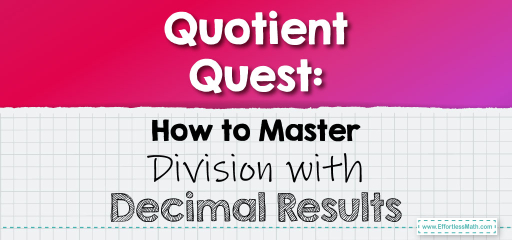# Quotient Quest: How to Master Division with Decimal Results

The division is one of the fundamental operations in mathematics. When both the dividend and divisor are whole numbers, the result can sometimes be a decimal. This is known as a decimal quotient. Let's dive into the process of division that results in decimal quotients.The Process Explained:

When dividing and the dividend isn’t completely divisible by the divisor, we introduce a decimal point in the quotient and continue the division process using the rules of decimal division.

## Dividing Using Decimal Quotients

### Example 1:

Divide $$5$$ by $$3$$.

Solution Process:

When you divide $$5$$ by $$3$$, you get a quotient of $$1$$ with a remainder of $$2$$. To continue the division, introduce a decimal point and add a zero to the right of the dividend. Now, divide $$20$$ by $$3$$.

The quotient is $$1.666…$$ (and so on). For simplicity, we can round it to $$1.67$$.

The Absolute Best Book for 5th Grade Students

### Example 2:

Divide $$7$$ by $$4$$.

Solution Process:

When you divide $$7$$ by $$4$$, you get a quotient of $$1$$ with a remainder of $$3$$. Introduce a decimal point in the quotient and add a zero to the right of the dividend. Now, divide $$30$$ by $$4$$.

The quotient is $$1.75$$.

Dividing to get decimal quotients is a valuable skill that broadens our understanding of division. It’s essential to remember that when the dividend isn’t completely divisible by the divisor, the division can continue into the decimal places. By mastering this concept, you’ll be better equipped to handle a wide range of mathematical problems, from everyday calculations to complex mathematical challenges. So, embrace the world of decimal quotients and elevate your division game!

### Practice Questions:

1. Divide $$8$$ by $$3$$.

2. Determine the quotient of $$11$$ divided by $$4$$.

3. Divide $$9$$ by $$5$$.

4. Determine the quotient of $$13$$ divided by $$6$$.

5. Divide $$15$$ by $$8$$.

A Perfect Book for Grade 5 Math Word Problems!

1. $$2.67$$ (rounded to two decimal places)

2. $$2.75$$

3. $$1.8$$

4. $$2.17$$ (rounded to two decimal places)

5. $$1.875$$

The Best Math Books for Elementary Students

### What people say about "Quotient Quest: How to Master Division with Decimal Results - Effortless Math: We Help Students Learn to LOVE Mathematics"?

No one replied yet.

X
51% OFF

Limited time only!

Save Over 51%

SAVE $15 It was$29.99 now it is \$14.99